## How to ace math exams simple tips and tricks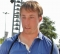Updated on 13-May-2022 06:47:07
In this article, you will learn simple tips and tricks to ace math exams on the fly. Although there are no shortcuts to success, focused study, regular practice of solving examples and worksheets, mastering the concepts are some of the best practices."If I were again beginning my studies, I would follow the advice of Plato and start with mathematics." Galileo GalileiStay Focused and Never Give-upWhen you study math, find a quiet place, get rid of the distractions, and focus on your work. You can easily make a mistake or miss a number otherwise.Understand and Master the ConceptsWhichever topic you are ... Read More

## Introduction to Algorithms for Mathematical Problems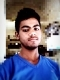Updated on 30-Jul-2019 22:30:23
In this section we will see some common mathematical problems and their possible way solve using different computational algorithms. We will see how to solve differential equations, integrations and some other complex mathematical problems. In this Section We are going to cover − Convert Infix to Postfix Expression Convert Infix to Prefix Expression Evaluate Postfix Expression Secant method to solve non-linear equation Trapezoidal Rule for definite integral Simpson's 1/3 Rule for definite integral Linear Regression Lagrange Interpolation Runge-Kutta 4th order rule for differential equation Lucky Numbers Decimal to Binary conversion Find LCM of two numbers Find ... Read More

## DFA based divisionUpdated on 17-Jun-2020 09:23:06
Deterministic Finite Automaton(DFA) is used to check whether a number is divisible by another number k or not. If it is not divisible, then this algorithm will also find the remainder.For the DFA based division, at first, we have to find the transition table of the DFA, using that table, we can easily find the answer. In the DFA, each state has only two transition 0 and 1.Input and OutputInput: The number: 50 and the divisor 3 Output: 50 is not divisible by 3 and remainder is: 2AlgorithmdfaDivision(num, k)Input: A number num, and divisor k.Output: Check divisibility and the remainder.Begin   ... Read More

## Find GCD of two numbers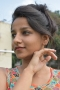Updated on 17-Jun-2020 08:18:25
In mathematics, Greatest Common Divisor (GCD) is the largest possible integer, that divides both of the integers. The condition is that the numbers must be non-zero.We will follow the Euclidean Algorithm to find the GCD of two numbers.Input and OutputInput: Two numbers 51 and 34 Output: The GCD is: 17AlgorithmfindGCD(a, b)Input: Two numbers a and b.Output: GCD of a and b.Begin    if a = 0 OR b = 0, then       return 0    if a = b, then       return b    if a > b, then       return findGCD(a-b, b)   ... Read More

## Find LCM of two numbersUpdated on 17-Jun-2020 08:19:33
In mathematics Least Common Multiple (LCM) is the smallest possible integer, that is divisible by both numbers.LCM can be calculated by many methods, like factorization, etc. but in this algorithm, we have multiplied the bigger number with 1, 2, 3…. n until we find a number which is divisible by the second number.Input and OutputInput: Two numbers: 6 and 9 Output: The LCM is: 18AlgorithmLCMofTwo(a, b)Input: Two numbers a and b, considered a > b.Output: LCM of a and b.Begin    lcm := a    i := 2    while lcm mod b ≠ 0, do       lcm := ... Read More

## Decimal to Binary conversion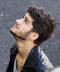Updated on 17-Jun-2020 08:24:51
A decimal number can also be converted into its binary form. To convert a decimal number to binary number, we need to divide the number by 2 until it reaches 0 or 1. And in each step, the remainder are stored separately to form the binary equivalent number in reverse order.In this algorithm, we will follow the recursive approach. It will help us to solve the problem without using stack data structure. In the implementation, we know that recursion of a function will follow the internal stack. We will serve our job by using that stack.Input and OutputInput: Decimal number ... Read More

## Lucky NumbersUpdated on 17-Jun-2020 08:23:49
Lucky numbers are some special integer numbers. From basic numbers, some special numbers are eliminated by their position. Instead of their value, for their position, the numbers are eliminated. The numbers which are not deleted, they are the lucky numbers.The number deletion follows some rule. At first, every second number are deleted, after that, all 3rd numbers are deleted and so on.Here is some example −1 2 3 4 5 6 7 8 9 10 11 12 13 14 15 16 17 18 19 20 21 22 23 24 25 (1 – 25 all) 1 3 5 7 9 11 ... Read More

## Lagrange Interpolation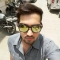Updated on 17-Jun-2020 08:34:36
For constructing new data points within a range of a discrete set of given data point, the interpolation technique is used. Lagrange interpolation technique is one of them. When the given data points are not evenly distributed, we can use this interpolation method to find the solution. For the Lagrange interpolation, we have to follow this equation.Input and OutputInput: List of x and f(x) values. find f(3.25) x: {0, 1, 2, 3, 4, 5, 6} f(x): {0, 1, 8, 27, 64, 125, 216} Output: Result after Lagrange interpolation f(3.25) = 34.3281AlgorithmlargrangeInterpolation(x: array, fx: array, x1)Input − x array and fx ... Read More

## Runge-Kutta 4th order rule for differential equationUpdated on 17-Jun-2020 08:37:44
Runge Kutta method is used for solving ordinary differential equations (ODE). It uses dy/dx function for x and y, and also need the initial value of y, i.e. y(0). It finds the approximate value of y for given x. For solving ODE, we have to follow these formulas:Here h is the height of the interval.Note: From these formulas, we can use first two k1 and k2 find the Runge-Kutta 2nd Order solution for ODE.Input and OutputInput: The x0 and f(x0): 0 and 0 the value of x = 0.4 the value of h = 0.1 Output: Answer of differential equation: ... Read More

## Linear RegressionUpdated on 17-Jun-2020 08:47:12
From a given set of data points, the linear regression finds an equation of straight line. The given points will follow the straight line. Using this formula, we can predict what will be the value for some other specific point, which is not present in the set currently.For solving linear regression problems using some data points, we have to follow these formulae:Here the m and c are the slope and the y-intercept respectively. Using these expressions, we can get the equation of straight line in this form: 𝑦 = 𝑚𝑥 + 𝑐.Input and OutputInput: The (x, y) coordinates of some ... Read More